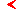mainscience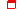this page only

# Critical behavior associated with quasiperiodicity at the golden-mean frequency ratio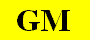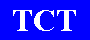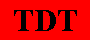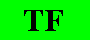## TF - Torus Fractalizationalphabet

Description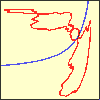This type of critical behavior occurs in two-parameter analysis of 1D maps locally approximated by fractional-linear map and forced with the golden-mean frequency. The critical point separates the situations of smooth and fractal tori-collision on the bifurcation curve.

RG equation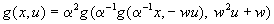The fixed point of the RG equation in class of fractional-linear maps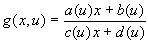,
a(u)=3.1801-3.4507u-3.2471u2
b(u)=0.3299-0.0030u-8.3305u2
c(u)=-1-0.6015u+0.4438u2
d(u)=0.2107+0.1672u+3.0628u2

The orbital scaling factors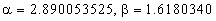Relevant eigenvalues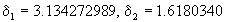Codimension
CoDim=2
(For 1D map, and, presumably, for more general systems too)

An example: the forced map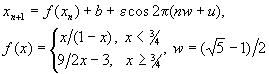The critical point is located at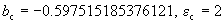Parameter space arrangement

Scaling coordinates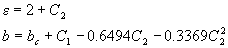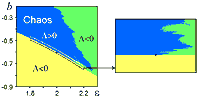show enlarged figure

Critical attractor: scaling withScaling coordinates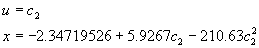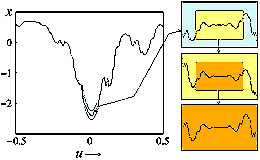show enlarged figuremainsciencealphabetthis page only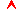top

Saratov group
of theoretical nonlinear
dynamics
Хостинг от uCoz